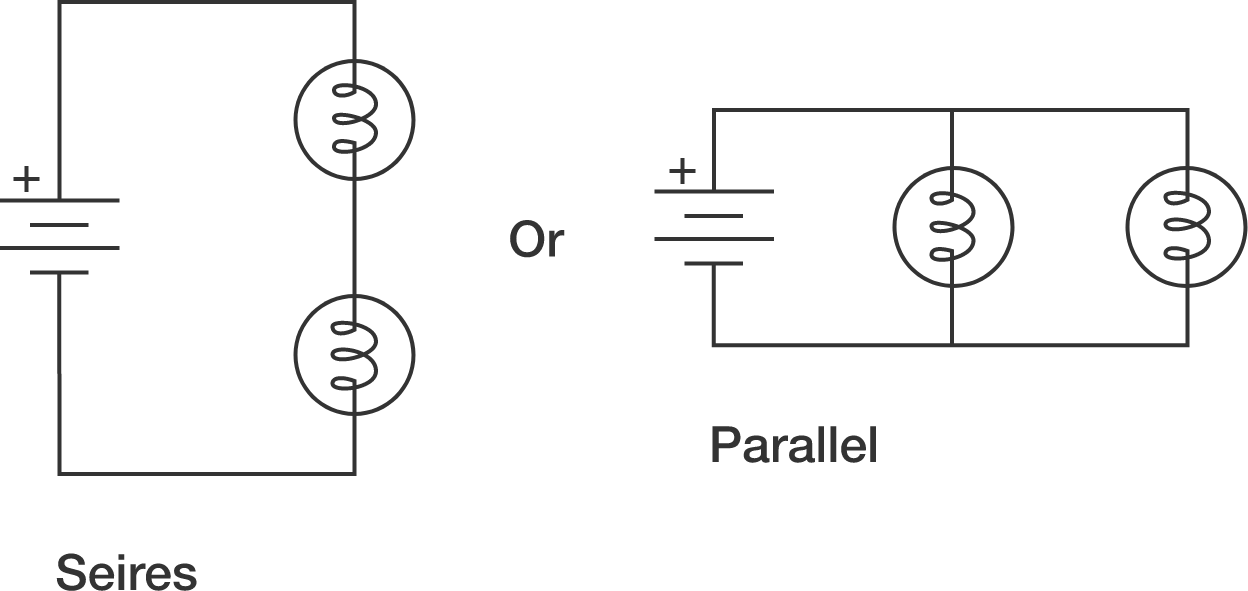# Series or parallel?

Jane hosted a party in her house but, unfortunately, the fuse has blown. She has an ideal battery (with zero resistance) and two bulbs. To produce a greater brightness (greater total power by the bulbs), should she connect the bulbs in series or in parallel with the battery?Note:

• The equivalent resistance $(R_{\text{net}})$ of two resistors $R_1$ and $R_2$ in series is $R_1+R_2,$ and in parallel $\frac{R_1R_2}{R_1+R_2}$.
• The power produced in a circuit is $\frac{V^2}{R_{\text{net}}}$ or $i^2 R_{\text{net}}$.
×

Problem Loading...

Note Loading...

Set Loading...